# Knowledge Base

## Solution 22610: Calculating One-Variable Statistics Using the TI-Nspire™ Family Products.

### How do I calculate one-variable statistics using the TI-Nspire family handheld or computer software?

To calculate one-variable statistics using the TI-Nspire family handheld or computer software, please follow the example listed below.

Example data: {1, 2, 5, 8}

Using the handheld:

2) In column "A", input the example data pressing [enter] after each entry.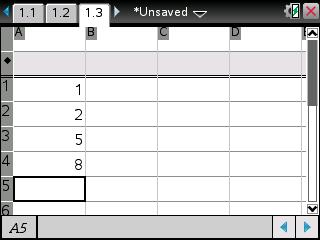3) Press [menu]   .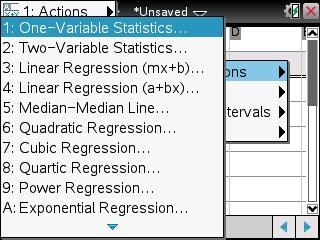4) When prompted for the "Num of Lists:", input "1" and press [enter].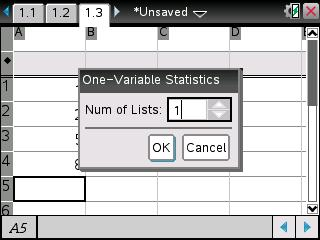5) In the "X1 List:" option box, input a[].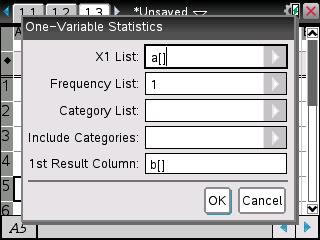6) Press [enter] to confirm the input and [enter] again to calculate the one-variable statistics of column A.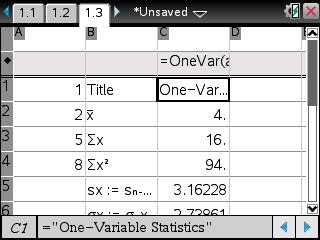Please Note: If the calculated data cannot be read (due to the column width), resize each column by first moving the cursor into the column to be expanded by pressing [menu]   .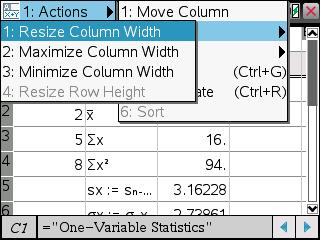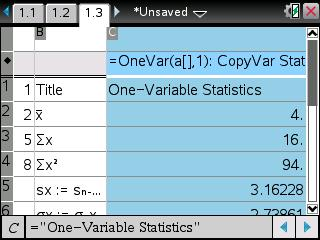Using TI-Nspire software: